GeeksforGeeks App
Open AppBrowser
Continue

## Related Articles

• CBSE Class 11 Physics Notes

Thermodynamics has become an integral element of our daily lives. Whether you’re in a car, sitting comfortably in an air-conditioned room, or sipping a cold beverage from the refrigerator, thermodynamics is used practically everywhere, either directly or indirectly.

When “Sadi Carnot” the father of thermodynamics, introduced thermodynamic theorems and cycles, few could have predicted that his ideas would one day play such a pivotal role in the invention of the vehicle, which has become an essential part of our lives. The thermodynamic cycles were further developed by Sterling Diesel, Otto, and Ericson, resulting in more inventions and improvements in automobiles. Before heading towards the Thermodynamic processes first we’ll see some concepts related to thermodynamics.

### Thermodynamics

The branch of science that deals with the transfer of energy from one form to another, as well as the relationship between heat and temperature, energy, and work done, is known as thermodynamics.

In other words, thermodynamics is the branch of science concerned with the study of the combined effects of heat and work on changes in the state of matter governed by thermodynamic principles.

The laws of thermodynamics are used to transfer heat energy linked with chemical reactions into different useable forms. Energy transformation is based on the fact that energy can only be transformed from one form to another and used in many industries. Chemical reactions have energy linked with them, as we all know. The laws of thermodynamics are concerned with energy changes that occur during a reaction, not with the pace at which the reaction occurs.

Importance of Thermodynamics

• It aids in determining whether or not a chemical reaction can occur under a certain set of conditions.
• It aids in forecasting the severity of a reaction.

### Thermodynamic System and the Surrounding

A system is defined in thermodynamics as the region of the universe that is being studied and where observations are being made. The environment and the universe interact with one another, and depending on the type of system, matter and energies are exchanged. The classification of a system is entirely dependent on the flow of energy and matter into and out of it. There are two different kinds of systems:

1. Open System-An open system is defined as one in which energy and matter are exchanged. When water is boiled on a stove without being covered, the container acts as an open system since it receives heat energy from an outside source and the stuff discharged is water vapors.
2. Closed System- A closed system is one in which only energy, rather than matter, can be exchanged with the environment. A closed system can alternatively be described as having a constant amount of matter, with the only variable being the system’s energy. When we keep a shield bottle of water in the fridge, for example, the loss of energy to the environment causes the temperature of the water inside the bottle to drop, despite the fact that the quantity of water in the bottle remains constant.
3. Isolated System- When a system is isolated, no energy nor matter can be exchanged between it and the environment. A Thermos flask is an example of an isolated system.

### First Law of Thermodynamics

The first law of thermodynamics is the universal law of energy conservation that applies to all systems. “The total heat energy change in every system is the sum of the internal energy change and the work done,” says this law. When a certain amount of heat, dQ, is applied to a system, a portion of it is used to increase internal energy, dU, and a portion is utilized to perform external work, dW, resulting in dQ = dU + dW.

The specific heat capacity of gases is determined by the procedure or conditions in which heat capacity is transferred. For gas, there are primarily two types of specific heat capacities. Specific heat capacity at constant volume and specific heat capacity at constant pressure are the two types of specific heat capacity.

We can identify a relationship between two primary specific temperatures of an ideal gas using the First Law of Thermodynamics. Cp-C = R, according to the relationship. The molar specific temperatures Cp and C are calculated under constant pressure and constant volume conditions, respectively.

Cp > C indicates that a gas’s specific heat capacity at constant pressure is greater than its specific heat capacity at constant volume. The reason for this is because when heat is delivered to a gas at a constant volume, the gas does no work against the external pressure, and all of the energy is used to raise the gas’s temperature. When heat is applied to gas at constant pressure, the volume of the gas rises, and the heat energy is used to raise the temperature of the gas as well as to perform work against the external pressure. The thermal equivalent of the effort done in expanding the gas against the external pressure is the difference between the two specific heats.

### Thermodynamic Processes

Let’s discuss the following Thermodynamic Processes in depth as follows:

1. Quasi-static Processes

The divergence of a system from its thermodynamic equilibrium in a quasistatic process is infinitesimally small. Equilibrium states are all the states that a system passes through throughout a quasi-static operation.

Consider a system in which gas is enclosed in a cylinder with a moving piston; if the piston is pushed at an endlessly slow rate, the system will remain in a state of quiescence all the time, and the process can be classified as quasi-static.

The process’s vanishingly slowness is a key property of quasi-static processes. During a quasi-static process, the system is always infinitesimally close to thermodynamic equilibrium. The concept of a quasi-static process is idealistic, and its conditions can never be met precisely in fact. In practice, processes that are sufficiently slow and do not require accelerated piston motion, a large temperature gradient, or other such factors are a good approximation to an ideal quasi-static process. Unless otherwise noted, the processes below exclusively define quasi-static processes.

2. Isothermal Process

The temperature of the system remains constant throughout an isothermal process.

Boyle’s law states that the pressure of a given mass of gas varies inversely with its volume when PV=constant. There is no change in temperature in an isothermal process since internal energy for an ideal gas depends only on temperature, hence there is no change in internal energy. As a result, U=0, and Q=W follows from the First Law of Thermodynamics. As a result, heat added (or withdrawn) from the system equals work done by (or on) the system during the isothermal process. Below is a PV diagram for the isothermal process.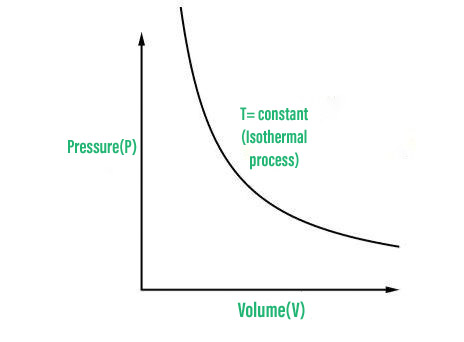PV graph

An adiabatic process is one in which no heat enters or leaves a system. For every adiabatic process: ΔQ=0.

Heat flow can be prevented by covering the system with a thick layer of heat-insulating material such as cork or asbestos. Heat flow takes a certain amount of time, so if a process is completed rapidly, it will be essentially adiabatic. When we apply the first law to an adiabatic process, we get,

ΔU = U2 −U1 = − Δ W

This pertains to the adiabatic process. The change in internal energy of a system in an adiabatic process is proportional to the work done by the system. When work is done on the system, it contracts, and  ΔW becomes negative. and the system’s internal energy grows by the same amount as the work done on it, and the system’s temperature rises.

If the system performs the task, ΔW is negative. The internal energy of systems reduces, resulting in a temperature drop. The relationship between Pressure and Volume in an adiabatic process with ideal gas is given by,

PV = γ = Constant

where γ=Cp/Cv. If an ideal gas changes state adiabatically from (P1, V1) to (P2, V2):

P1V1=P2V2

PV diagram for the adiabatic is,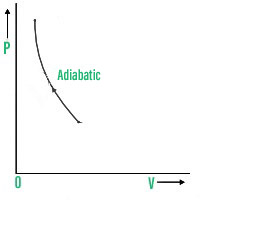PV graph

4. Isochoric Process

The volume of the system remains uncharged during an isochoric phase, i.e. ΔV = 0.

When the volume does not change, no work is done, ΔW = 0, and so the first law of thermodynamics is violated.

U2-U1 = ΔU = ΔQ

All of the heat delivered to the system has been used to boost the system’s internal energy. The equation linking P, V, and T in an isochoric process yields , P/T=constant i.e. as the temperature rises, the pressure rises. PV diagram of the isochoric process.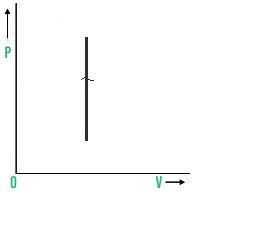PV graph

5. Isobaric Process

Isobaric process refers to a process that occurs at constant pressure.

We can see from this equation that the work done in the isobaric process is W=P(V2-V1)= n R(T2-T1) where the pressure is maintained at a consistent level. The amount of heat supplied to the system is split between rising temperature and doing work in this process i.e.

ΔQ = ΔU + ΔW

The equation linking P, V, and T for an isobaric process is V/T=constant i.e. as the temperature rises, the volume rises. The PV diagram of the isobaric process can be drawn as,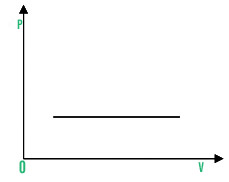PV graph

Cyclic Process

The system returns to its beginning condition in a cyclic process. Since the system has returned to its starting state, there has been no change in internal energy ΔU=0. Here in this process total heat absorbed is equal to total work done by the system i.e.

ΔQ = ΔW

The PV diagram of the cyclic process can be drawn as,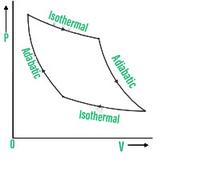PV graph

### Sample Problems

Problem 1: Calculate the number of kilojoules of heat required to raise the temperature of 60 g of aluminium from 35 to 55 degrees Celsius. Aluminium has a molar heat capacity of 24 J mol-1 K-1

Solution:

No. of moles of Al (m) = (60 g)/(27 g mol-1) = 2.22 mol

Molar heat capacity (C) = 24  J mol-1 K-1.

Rise in temperature (∆T) = 55 – 35 = 20 °C = 20 K

Heat evolved (q) = C x m x T = (24 J mol-1 K-1) x (2.22 mol) x (20 K)

= 1065.6 J

Problem 2: For an isolated system ∆U = 0; what will be ∆S?

Solution:

An isolated system has no change in internal energy (∆U) since it does not exchange energy with its surroundings. In the event of a spontaneous reaction, however, entropy tends to grow. As a result, ∆S > 0 or positive.

Problem 3: Write the limitations of Thermodynamics.

Limitations of Thermodynamics are;

• It aids in the prediction of a process’s feasibility but does not provide information on the rate of reaction.
• It merely discusses a system’s initial and final states, but it doesn’t go into detail regarding the process’ mechanism.

Problem 4: Define the first law of motion?

Solution:

Heat is a kind of energy, according to the First Law of Thermodynamics, and thermodynamic processes are therefore subject to the concept of energy conservation. Heat energy cannot be created or destroyed in this way. It can, however, be moved from one spot to another and changed into and out of different types of energy.

The first law of thermodynamics has the following equation:

ΔU = q + W

where,

• ΔU is the change in the system’s internal energy.
• q denotes the algebraic sum of heat transmission between the system and the environment.
• W stands for the system’s work relationship with its environment.

Problem 5: In a system, the pressure of a gas remains constant. A total of 45 J of heat is lost in the environment around the system. The system receives 450 J of work. What is the internal energy of the system?

Solution:

We know that equation of first law of motion;

ΔU = q+ w

ΔU = 45J + 450J

ΔU = 495J

My Personal Notes arrow_drop_up
Related Tutorials